# Function

This page lists a core term of calculus. The term is used widely, and a thorough understanding of its definition is critical.
See a complete list of core terminology

## Definition

### General definition

A function from a set$A$ to a set$B$ is a rule that associates, to each element of$A$, a unique element of$B$.

Functions are typically denoted by lower-case or upper-case single letters, though some functions have special notations. To say that a function$f$ is from$A$ to$B$, we write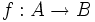$f:A \to B$ is a function.

For an element$a$ of the domain$A$, the unique element of$B$ associated with$a$ is denoted as$f(a)$. The act of going from$a$ to$f(a)$ is termed applying the function. The element$a$ is termed an input to the function and the corresponding element$f(a)$ is termed the output or image of the function corresponding to that input.

Note that$A$ and$B$ may be equal or distinct.

Some key terminology:

• The domain of a function$f:A \to B$ is the set$A$.
• This term is not used in most basic treatments of the calculus of one variable: The co-domain of a function$f:A \to B$ is the set$B$.
• The range of a function$f:A \to B$ is the subset of$B$ given as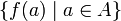$\{ f(a) \mid a \in A \}$, i.e., the set of elements of$B$ that arise as outputs of the function.

### Definition for functions of one variable

In the context of functions of one variable, the term function is used for a function whose domain is a subset of$\R$ and whose co-domain is$\R$, i.e., for a real-valued function with a real variable as input. In other words, the term function is used for a function if both the domain and the range are subsets of$\R$.

## Key features

### Equal inputs give equal outputs

A key feature of functions is that, for a given function, the input to the function completely determines the output, i.e., if the same input is fed into the same function at different times, the output will be the same each time. This feature can be captured by the phrase equal inputs give equal outputs.

When people ask whether a function is well defined, what they usually mean is whether it is a function at all, in the sense of whether it has the key feature of equal inputs give equal outputs.

Here are some examples:

Example of a purported function Purported domain Does it satisfy the equal inputs give equal outputs property? So, is it really a function?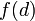$f(d)$ is defined as the area of a circle with diameter$d$ Positive reals Yes, because any two circles with the same diameter are congruent and hence have the same area. In fact, we can also obtain an explicit formula: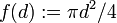$f(d) := \pi d^2/4$ Yes$f(x)$ is defined as the area of a square with perimeter$x$ Positive reals Yes, because any two squares with the same perimeter are congruent and hence have the same area. In fact, we can also obtain an explicit formula: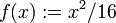$f(x) := x^2/16$ Yes$f(x)$ is defined as the area of a rectangle with perimeter$x$ Positive reals No, because different rectangles with the same perimeter can have different shapes and consequently have different areas No

### Domain and restriction of domain

The study of a function depends crucially on the domain on which the function is being studied. If a function of one variable is defined solely by means of an expression or procedure, the domain of the function is taken to be the largest possible subset of the reals on which that expression or procedure makes sense and gives a valid answer. However, we can also consider functions restricted to domains that are strictly smaller than the maximum possible domain on which the expression being used for the function makes sense. The behavior of the function, as well as answers to questions like whether it is increasing or decreasing and what its extreme values are, depends on what domain we are considering the function on.

Here are some examples:

• Consider the function$f$ defined as follows:$f(d)$ is defined as the area of a circle with diameter$d$. Ignoring the boundary case of point circles and line circles, the only possible inputs for this function are positive reals, so$f$ is a function from the positive reals to the positive reals given by the expression$f(d) := \pi d^2/4$. However, if we look only at the expression for$f$, then that expression makes sense for all real numbers, including zero and negative real numbers as well as positive real numbers. Call the latter function$g$, i.e.,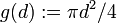$g(d) := \pi d^2/4$ for all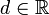$d \in \R$. Then,$f$ is the restriction of$g$ to the subdomain$(0,\infty)$. Note that:
•$g$ is not an increasing function, but the restriction$f$ is an increasing function.
•$g$ is not a one-one function, but the restriction$f$ is a one-one function.
•$g$ attains its absolute minimum value, but the restriction$f$ does not.

## Description of functions

A description of a function should be a clear and actionable way of describing (i) what the domain is, and (ii) how to compute the output of the function from any given input in the domain. There are three main kinds of descriptions:

• Algebraic (expression-based or formula-based, or procedural)
• Numerical (in the form of a table of inputs and outputs)
• Graphical (in the form of the graph of the function)

### Algebraic, expression-based or procedural descriptions

This is a description of the function that uses a formula or expression based on known functions and known techniques for pointwise combination, composition, taking the inverse function, and using piecewise definitions. The known techniques are the tools used to build complicated functions from the existing simpler ones.

An algebraic description is typically written in the form:$\! x \mapsto$ expression in$x$

The way this is interpreted is that, to evaluate the function at any actual input, we replace all occurrences of$x$ on the right side with that input and compute.

Alternatively, if the function is denoted by the letter$g$, we can also write, in place of the above:$\! g(x) :=$ expression in$x$

DUMMY VARIABLE: The letter$x$ used above is a dummy variable (or a local variable). In other words, replacing$x$ on both the left side and in the right side expression by a single other letter gives an identical function definition. Also, if the letter$x$ is already in use, then some other letter should be used for the function description.

In some cases, the algebraic description may be too complicated to write in a single straight line expression. In this case, we may express it in terms of a procedure or algorithm.

Here are some examples:

Function Algebraic description of function Comments
identity function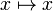$x \mapsto x$
square function$x \mapsto x^2$
sine-squared function$x \mapsto \sin^2x$ We are using a composite of two functions: the square function and the sine function.
absolute value function $array$ we use a piecewise definition of function. Specifically, this function is a piecewise linear function.